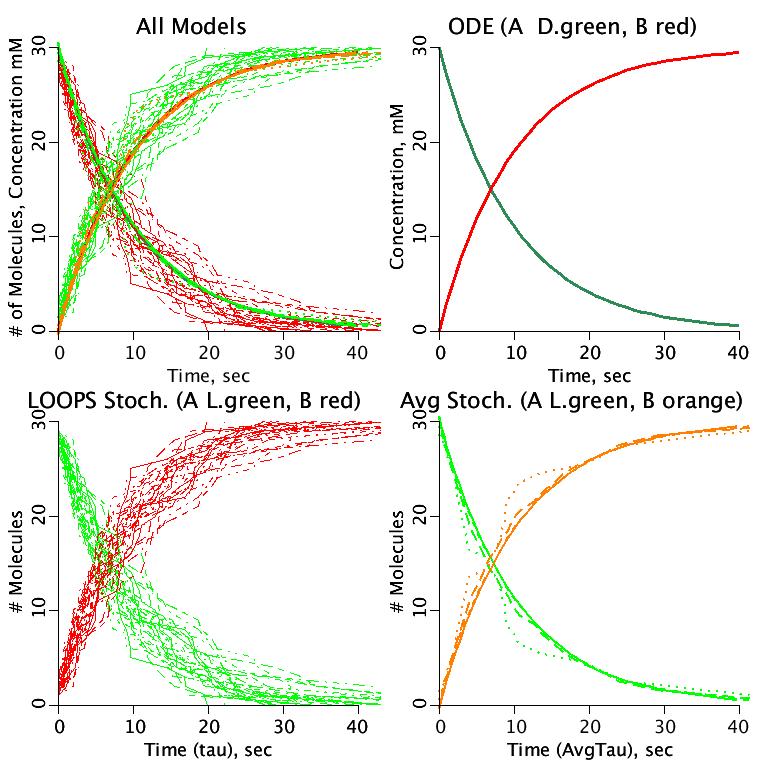# StochasticReaction

Model number
0328

A reaction, A -> B with rate constant k is modeled using an ordinary differential equation and Gillespie's stochastic method.

## Description

```Reaction kinetics with a finite number of molecules.
This project file contains 3 separate models.

Model 1: ODE_Reaction computes the exponential decay of substance
A producing substance B use Ordinary Differential Equations.

Model 2: Stochastic_Reaction computes the decay of 30 molecules of A
producing 30 molecules of B. The Time between decay events is controlled
by a random exponential distribution ( see Gillespie, 1977). Either
Single Run or Loops Run to see envelope of stochastic solutions.

Model 3: Average_Stochastic_Time computes the average time for the
number of remaining molecules of A compared to the ODE_Reaction curves.
A thousand curves are generated and the average time as a function of the
molecules remaining is calculated. These curves are compared with the ODE
model.

Explanation of Gillespie's Stochastic Method:

Compute lambda1 as shown in the code.
Sum the lambda's to calculate lambda.
Create a random exponential distribution by taking the natural
log of uniform random numbers.
When multiplied by -1/lambda it becomes the time step when
another reaction takes place. The uneven time steps are
accumulated in tau.
A second uniform random number for which reaction takes place
is determined by the probability of the reaction which is the
ratio of its lambda to the sum of all the other lambdas.
We Calculate P1=lambda1/lambda, P2=(lambda1+lambda2)/lambda, ...
For the reaction selected, the appropriate pools are
incremented or decremented in whole units.

NOTE: to increase the number of molecules by a factor
of 10, divide k1 by 10. Be sure to make the corresponding
change in the ODE model.
```## Equations

The equations for this model may be viewed by running the JSim model applet and clicking on the Source tab at the bottom left of JSim's Run Time graphical user interface. The equations are written in JSim's Mathematical Modeling Language (MML). See the Introduction to MML and the MML Reference Manual. Additional documentation for MML can be found by using the search option at the Physiome home page.

References
```D.T. Gillespie, Exact stochastic simulation of coupled chemical reactions,
J. Phys. Chem., 1977, 81 (25), pp 2340–2361.```
Key terms
Reaction
ODE
Stochastic
Gillespie
Acknowledgements

Please cite https://www.imagwiki.nibib.nih.gov/physiome in any publication for which this software is used and send one reprint to the address given below:
The National Simulation Resource, Director J. B. Bassingthwaighte, Department of Bioengineering, University of Washington, Seattle WA 98195-5061.

Model development and archiving support at https://www.imagwiki.nibib.nih.gov/physiome provided by the following grants: NIH U01HL122199 Analyzing the Cardiac Power Grid, 09/15/2015 - 05/31/2020, NIH/NIBIB BE08407 Software Integration, JSim and SBW 6/1/09-5/31/13; NIH/NHLBI T15 HL88516-01 Modeling for Heart, Lung and Blood: From Cell to Organ, 4/1/07-3/31/11; NSF BES-0506477 Adaptive Multi-Scale Model Simulation, 8/15/05-7/31/08; NIH/NHLBI R01 HL073598 Core 3: 3D Imaging and Computer Modeling of the Respiratory Tract, 9/1/04-8/31/09; as well as prior support from NIH/NCRR P41 RR01243 Simulation Resource in Circulatory Mass Transport and Exchange, 12/1/1980-11/30/01 and NIH/NIBIB R01 EB001973 JSim: A Simulation Analysis Platform, 3/1/02-2/28/07.